Chapter 3: Graphing

# 3.4 Graphing Linear Equations

There are two common procedures that are used to draw the line represented by a linear equation. The first one is called the slope-intercept method and involves using the slope and intercept given in the equation.

If the equation is given in the form $y = mx + b$, then $m$ gives the rise over run value and the value $b$ gives the point where the line crosses the $y$-axis, also known as the $y$-intercept.

Example 3.4.1

Given the following equations, identify the slope and the $y$-intercept.

1. $\begin{array}{lll} y = 2x - 3\hspace{0.14in} & \text{Slope }(m)=2\hspace{0.1in}&y\text{-intercept } (b)=-3 \end{array}$
2. $\begin{array}{lll} y = \dfrac{1}{2}x - 1\hspace{0.08in} & \text{Slope }(m)=\dfrac{1}{2}\hspace{0.1in}&y\text{-intercept } (b)=-1 \end{array}$
3. $\begin{array}{lll} y = -3x + 4 & \text{Slope }(m)=-3 &y\text{-intercept } (b)=4 \end{array}$
4. $\begin{array}{lll} y = \dfrac{2}{3}x\hspace{0.34in} & \text{Slope }(m)=\dfrac{2}{3}\hspace{0.1in} &y\text{-intercept } (b)=0 \end{array}$

When graphing a linear equation using the slope-intercept method, start by using the value given for the $y$-intercept. After this point is marked, then identify other points using the slope.

This is shown in the following example.

Example 3.4.2

Graph the equation $y = 2x - 3$.

First, place a dot on the $y$-intercept, $y = -3$, which is placed on the coordinate $(0, -3).$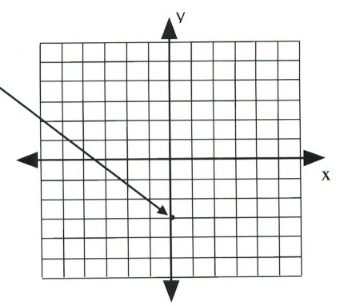Now, place the next dot using the slope of 2.

A slope of 2 means that the line rises 2 for every 1 across.

Simply, $m = 2$ is the same as $m = \dfrac{2}{1}$, where $\Delta y = 2$ and $\Delta x = 1$.

Placing these points on the graph becomes a simple counting exercise, which is done as follows: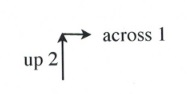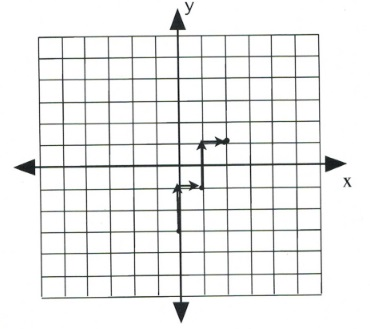Once several dots have been drawn, draw a line through them, like so: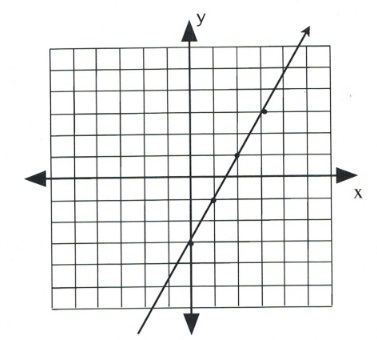Note that dots can also be drawn in the reverse of what has been drawn here.

Slope is 2 when rise over run is $\dfrac{2}{1}$ or $\dfrac{-2}{-1}$, which would be drawn as follows: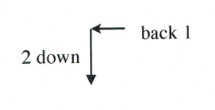Example 3.4.3

Graph the equation $y = \dfrac{2}{3}x$.

First, place a dot on the $y$-intercept, $(0, 0)$.

Now, place the dots according to the slope, $\dfrac{2}{3}$.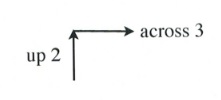This will generate the following set of dots on the graph. All that remains is to draw a line through the dots.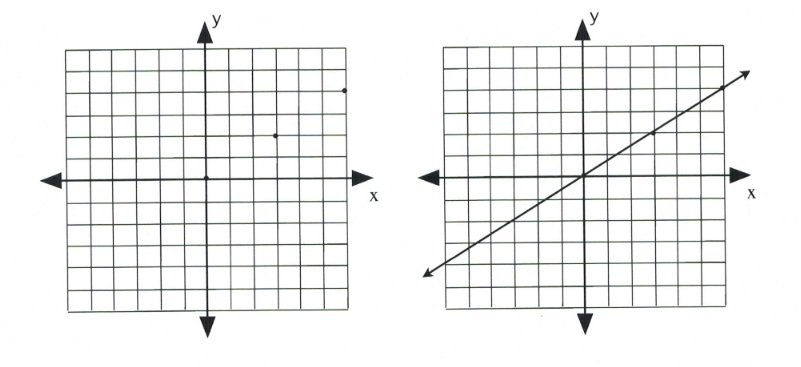The second method of drawing lines represented by linear equations and functions is to identify the two intercepts of the linear equation. Specifically, find $x$ when $y = 0$ and find $y$ when $x = 0$.

Example 3.4.4

Graph the equation $2x + y = 6$.

To find the first coordinate, choose $x = 0$.

This yields:

$\begin{array}{lllll} 2(0)&+&y&=&6 \\ &&y&=&6 \end{array}$

Coordinate is $(0, 6)$.

Now choose $y = 0$.

This yields:

$\begin{array}{llrll} 2x&+&0&=&6 \\ &&2x&=&6 \\ &&x&=&\frac{6}{2} \text{ or } 3 \end{array}$

Coordinate is $(3, 0)$.

Draw these coordinates on the graph and draw a line through them.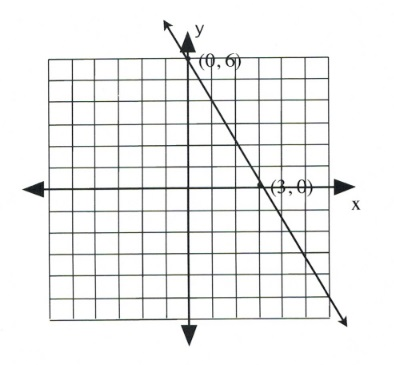Example 3.4.5

Graph the equation $x + 2y = 4$.

To find the first coordinate, choose $x = 0$.

This yields:

$\begin{array}{llrll} (0)&+&2y&=&4 \\ &&y&=&\frac{4}{2} \text{ or } 2 \end{array}$

Coordinate is $(0, 2)$.

Now choose $y = 0$.

This yields:

$\begin{array}{llrll} x&+&2(0)&=&4 \\ &&x&=&4 \end{array}$

Coordinate is $(4, 0)$.

Draw these coordinates on the graph and draw a line through them.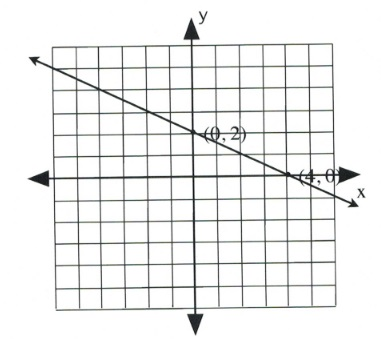Example 3.4.6

Graph the equation $2x + y = 0$.

To find the first coordinate, choose $x = 0$.

This yields:

$\begin{array}{llrll} 2(0)&+&y&=&0 \\ &&y&=&0 \end{array}$

Coordinate is $(0, 0)$.

Since the intercept is $(0, 0)$, finding the other intercept yields the same coordinate. In this case, choose any value of convenience.

Choose $x = 2$.

This yields:

$\begin{array}{rlrlr} 2(2)&+&y&=&0 \\ 4&+&y&=&0 \\ -4&&&&-4 \\ \hline &&y&=&-4 \end{array}$

Coordinate is $(2, -4)$.

Draw these coordinates on the graph and draw a line through them.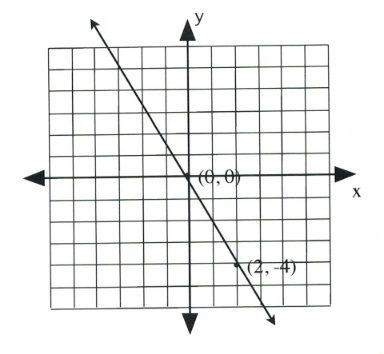# Questions

For questions 1 to 10, sketch each linear equation using the slope-intercept method.

1. $y = -\dfrac{1}{4}x - 3$
2. $y = \dfrac{3}{2}x - 1$
3. $y = -\dfrac{5}{4}x - 4$
4. $y = -\dfrac{3}{5}x + 1$
5. $y = -\dfrac{4}{3}x + 2$
6. $y = \dfrac{5}{3}x + 4$
7. $y = \dfrac{3}{2}x - 5$
8. $y = -\dfrac{2}{3}x - 2$
9. $y = -\dfrac{4}{5}x - 3$
10. $y = \dfrac{1}{2}x$

For questions 11 to 20, sketch each linear equation using the $x\text{-}$ and $y$-intercepts.

1. $x + 4y = -4$
2. $2x - y = 2$
3. $2x + y = 4$
4. $3x + 4y = 12$
5. $2x - y = 2$
6. $4x + 3y = -12$
7. $x + y = -5$
8. $3x + 2y = 6$
9. $x - y = -2$
10. $4x - y = -4$

For questions 21 to 28, sketch each linear equation using any method.

1. $y = -\dfrac{1}{2}x + 3$
2. $y = 2x - 1$
3. $y = -\dfrac{5}{4}x$
4. $y = -3x + 2$
5. $y = -\dfrac{3}{2}x + 1$
6. $y = \dfrac{1}{3}x - 3$
7. $y = \dfrac{3}{2}x + 2$
8. $y = 2x - 2$

For questions 29 to 40, reduce and sketch each linear equation using any method.

1. $y + 3 = -\dfrac{4}{5}x + 3$
2. $y - 4 = \dfrac{1}{2}x$
3. $x + 5y = -3 + 2y$
4. $3x - y = 4 + x - 2y$
5. $4x + 3y = 5 (x + y)$
6. $3x + 4y = 12 - 2y$
7. $2x - y = 2 - y \text{ (tricky)}$
8. $7x + 3y = 2(2x + 2y) + 6$
9. $x + y = -2x + 3$
10. $3x + 4y = 3y + 6$
11. $2(x + y) = -3(x + y) + 5$
12. $9x - y = 4x + 5$